### CYCLE Statement

CYCLE <options> ;

The CYCLE statement is used to specify a cycle component,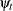, in the model. The stochastic equation governing a cycle component of period p and damping factor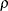is as follows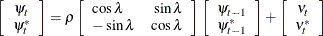where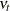and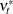are independent, zero-mean, Gaussian disturbances with variance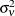and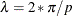is the angular frequency of the cycle. Any p strictly greater than two is an admissible value for the period, and the damping factorcan be any value in the interval (0, 1), including one but excluding zero. The cycles with frequency zero and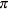, which correspond to the periods equal to infinity and two, respectively, can be specified using the AUTOREG statement. The values ofless than one give rise to a stationary cycle, while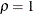gives rise to a nonstationary cycle. As a default, values of, p, andare estimated from the data. However, if necessary, you can fix the values of some or all of these parameters.

There can be multiple cycles in a model, each specified using a separate CYCLE statement. The examples that follow illustrate the use of the CYCLE statement.

The following statements request including two cycles in the model. The parameters of each of these cycles are estimated from the data.

      cycle;
cycle;


The following statement requests inclusion of a nonstationary cycle in the model. The cycle period p and the disturbance varianceare estimated from the data.

      cycle rho=1 noest=rho;


In the following statement a nonstationary cycle with a fixed period of 12 is specified. Moreover, a starting value is supplied for.

      cycle period=12 rho=1 variance=4 noest=(rho period);

NOEST=PERIOD
NOEST=RHO
NOEST=VARIANCE
NOEST=( <RHO> <PERIOD> <VARIANCE> )

fixes the values of the component parameters to those specified in the RHO=, PERIOD=, and VARIANCE= options. This option enables you to fix any combination of parameter values.

PERIOD=value

specifies an initial value for the cycle period during the parameter estimation process. Period value must be strictly greater than 2.

PLOT=FILTER
PLOT=SMOOTH
PLOT=( <FILTER> <SMOOTH> )

requests plotting of the filtered or smoothed estimate of the cycle component.

PRINT=FILTER
PRINT=SMOOTH
PRINT=( <FILTER> <SMOOTH> )

requests the printing of a filtered or smoothed estimate of the cycle component.

RHO=value

specifies an initial value for the damping factor in this component during the parameter estimation process. Any value in the interval (0, 1), including one but excluding zero, is an acceptable initial value for the damping factor.

VARIANCE=value

specifies an initial value for the disturbance variance parameter,, to be used during the parameter estimation process. Any nonnegative value, including zero, is an acceptable starting value.